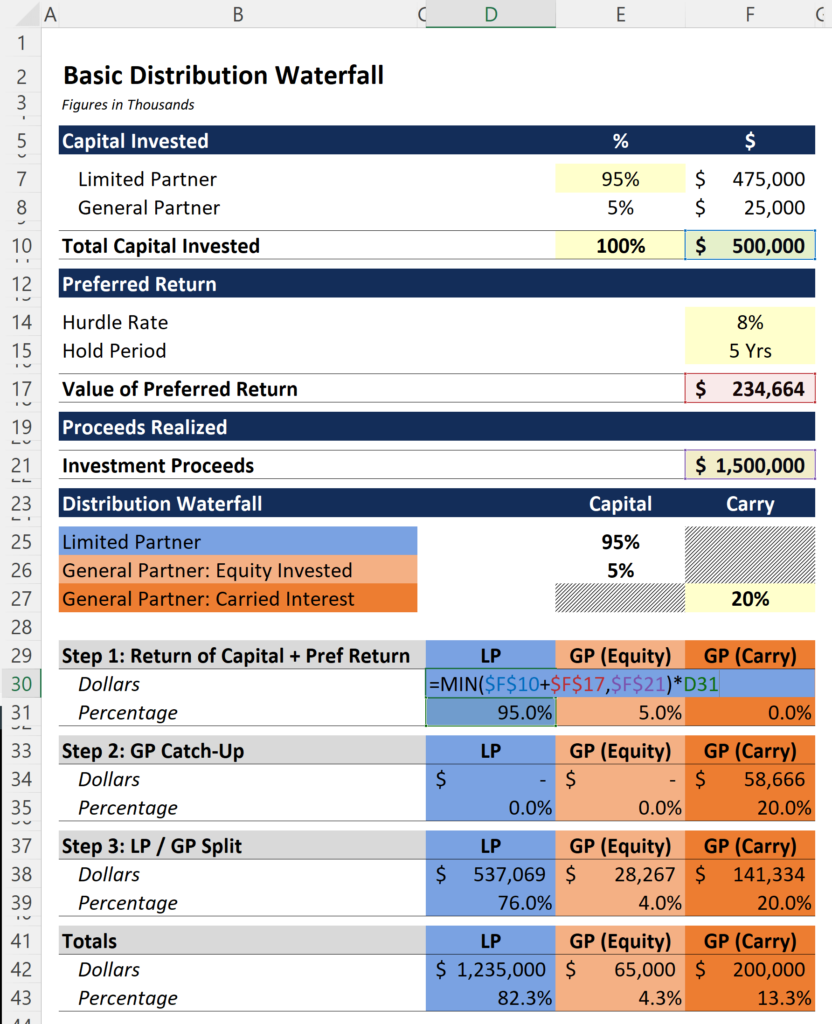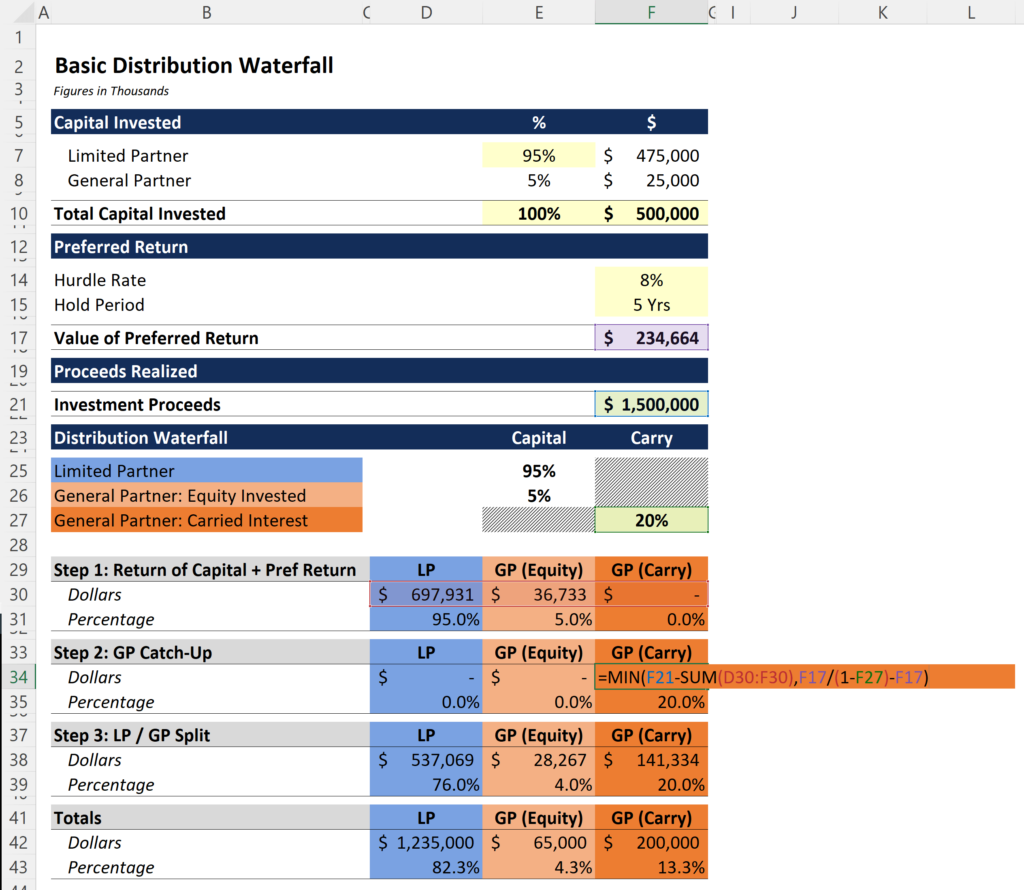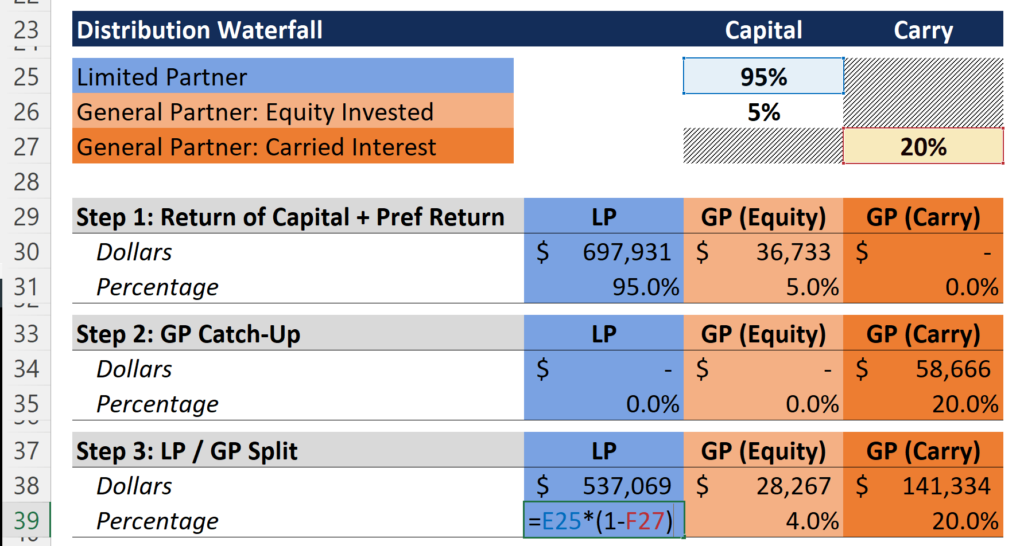Select Page

# Carried Interest for a \$500M Private Equity Fund

• Peter Lynch

How much carried interest would private equity fund managers earn on a \$500 million dollar fund that triples in value? In this post we will walk through a basic distribution waterfall to explain how this calculation works. (Note: This video moves quickly. A slower video for those following along in Excel is available at the bottom of this post.)

Private Equity Fund Assumptions:

• Fund Size: \$500 Million
• GP Invests 5% (“Skin in the Game”)
• Preferred Return: 8%
• Carried Interest: 20%
• Hold Period: 5 Years
• Investment Proceeds: \$1.5 Billion

Distribution Waterfall:

1. First, 100% of all cash inflows to the LP until the cumulative distributions equal the original capital invested plus the preferred return.
2. Second, a “20% catch-up” to the GP equivalent to 20% of the value of the preferred return plus the distributions realized in this step.
3. Third, thereafter, cash flows in excess of distributions made in step 1 and 2 (if any) are distributed 80% to the LP and 20% to the GP.

Step 1: The investors (or limited partners) must earn back their capital plus any preferred return before the GP can earn carried interest. Using the =MIN function, select the sum of the capital invested and the preferred return as the first value and the total investment proceeds as the second value. Then multiply this value by the pro rata contribution of the GP and the LP. Everything under “GP (Carry)” is zeroed out because the PE firm cannot earn carry at this stage (see image below).Step 2: The next step is to calculate the value of the catch up, which goes entirely to the PE firm in the form of carried interest. For this step, the array under “LP” and “GP (Equity)” should be zeroed out.

To calculate the amount of the catch up, we will again use the =MIN function to determine if we have enough proceeds left over after step 1, and compare this value to the value of the catch up. Inside the =MIN function we have two values: (1) investment proceeds less all distributions made in step 1, and (2) the value of the catch up. The value of the catch up is equal to the value of the preferred return divided by 80% less the value of the preferred return (formula explanation).Step 3: Now it gets much easier. The 80 / 20 split between the LP and the GP requires taking the remaining proceeds and multiplying by the appropriate pro rata percentage. Since the GP invested, we have to modify the percentages under “LP” and “GP (Equity)” by multiplying the original contribution by 80%. This dilutes both groups by the amount of the carry, which is equal to 20%. You can see this calculation in the first image below.Once the percentages have been calculated for each of the three categories, the remaining proceeds can be split according to these percentages. The formula subtracts all distributions made in steps 1 and 2 by the amount of investment proceeds and then multiplies this value by the percentage calculated in the image above (see below).The final step simply involves summing the values in steps 1, 2 and 3 to arrive at the total for each category. Once complete, you should have a carried interest equal to \$200 million. As an exercise, the template is available for download at the bottom of this post with a worksheet that includes the formatted cells but none of the formulas. Try to build it yourself!

Excel Template: Distribution Waterfall_Carried Interest_ASM